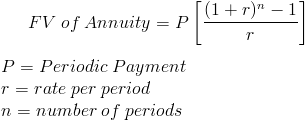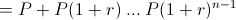# Future Value of AnnuityThe future value of an annuity formula is used to calculate what the value at a future date would be for a series of periodic payments.

The future value of an annuity formula assumes that

1. The rate does not change
2. The first payment is one period away
3. The periodic payment does not change

If the rate or periodic payment does change, then the sum of the future value of each individual cash flow would need to be calculated to determine the future value of the annuity. If the first cash flow, or payment, is made immediately, the future value of annuity due formula would be used.

## Example of Future Value of an Annuity Formula

An example of the future value of an annuity formula would be an individual who decides to save by depositing \$1000 into an account per year for 5 years. The first deposit would occur at the end of the first year. If a deposit was made immediately, then the future value of annuity due formula would be used. The effective annual rate on the account is 2%. If she would like to determine the balance after 5 years, she would apply the future value of an annuity formula to get the following equationThe balance after the 5th year would be \$5204.04.

## How is the Future Value of an Annuity Derived?

The future value of an annuity is the future value of a series of cash flows. The formula for the future value of an annuity, or cash flows, can be written asWhen the payments are all the same, this can be considered a geometric series with 1+r as the common ratio.

Using the geometric series formula, the future value of an annuity formula becomesThe denominator then becomes -r. The negative r in the denominator can be remedied by multiplying the entire formula by -1/-1, which is the same as multiplying by 1. This will return the formula shown on the top of the page.

New to Finance?